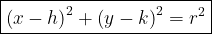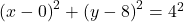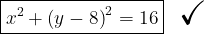## What is the equation of a circle with radius 4 and center (0,8)?

Question

What is the equation of a circle with radius 4 and center (0,8)?

in progress 0
6 months 2021-09-03T21:56:49+00:00 1 Answers 0 views 0

1. The equation of circle with the center at (h,k) is:The question has already given the information we need, which are:

• the center at (0,8)

Since the center is (h,k) – therefore the center is at h = 0 and k = 8 making it (h,k) = (0,8).

Substitute the values in the equation:Simplify to the simplest form